Browse by Topic

# A New Method of Bell Ringing Using Mathematica to Discover Wolf Wrap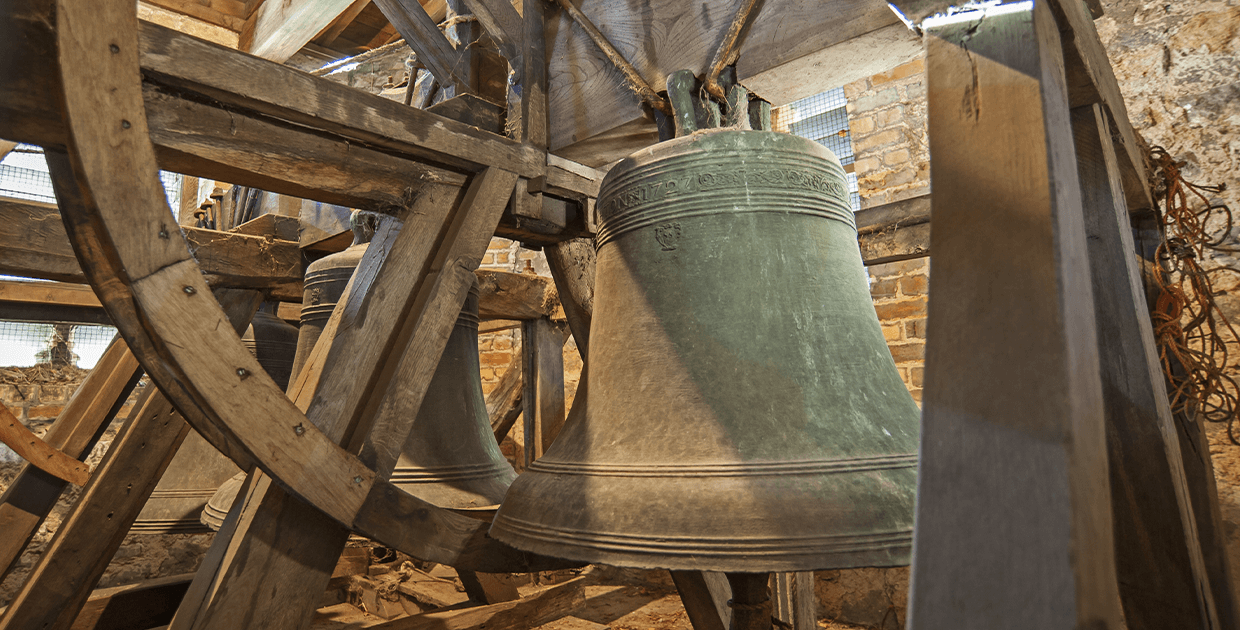English bell ringing (called change ringing) has many connections to mathematics, notably to group theory and Hamiltonian cycles. My wife, Joan Hutchinson, is an ardent bell ringer (having rung in both England and North America), and I knew the basics of this ancient craft. A recent puzzle book by Mark Davies  inspired me to bring Mathematica’s integer-linear programming (ILP) capabilities to bear, but I wanted to go beyond puzzles and develop a new ringing method that would be of interest to the bell-ringing community.

My idea of finding a method with a large number of “wraps” was a success, and the method, which I called Wolf Wrap, has now been rung by a British band of ringers; this means it was formally entered on the Ringing World’s list of recognized methods. Here I will describe how one can interpret the problem in terms of a linear system of inequalities and so apply ILP, which is accessed via Mathematica’s LinearOptimization function.

## 1. Bell-Ringing Basics

The English hang their bells in a unique way: each bell is attached to a wheel that rotates when a rope is pulled by the ringer. The wheel bell in the tower swings through a full revolution from mouth upward to mouth upward for each sounded tone. There is one ringer for each bell in the tower; when methods are rung on hand bells, each ringer controls two bells.

This is the “ringing chamber” at Stoke Gabriel Parish Church, Devon, England. The ringer pulls on the rope to turn the wheel to which the bell is attached.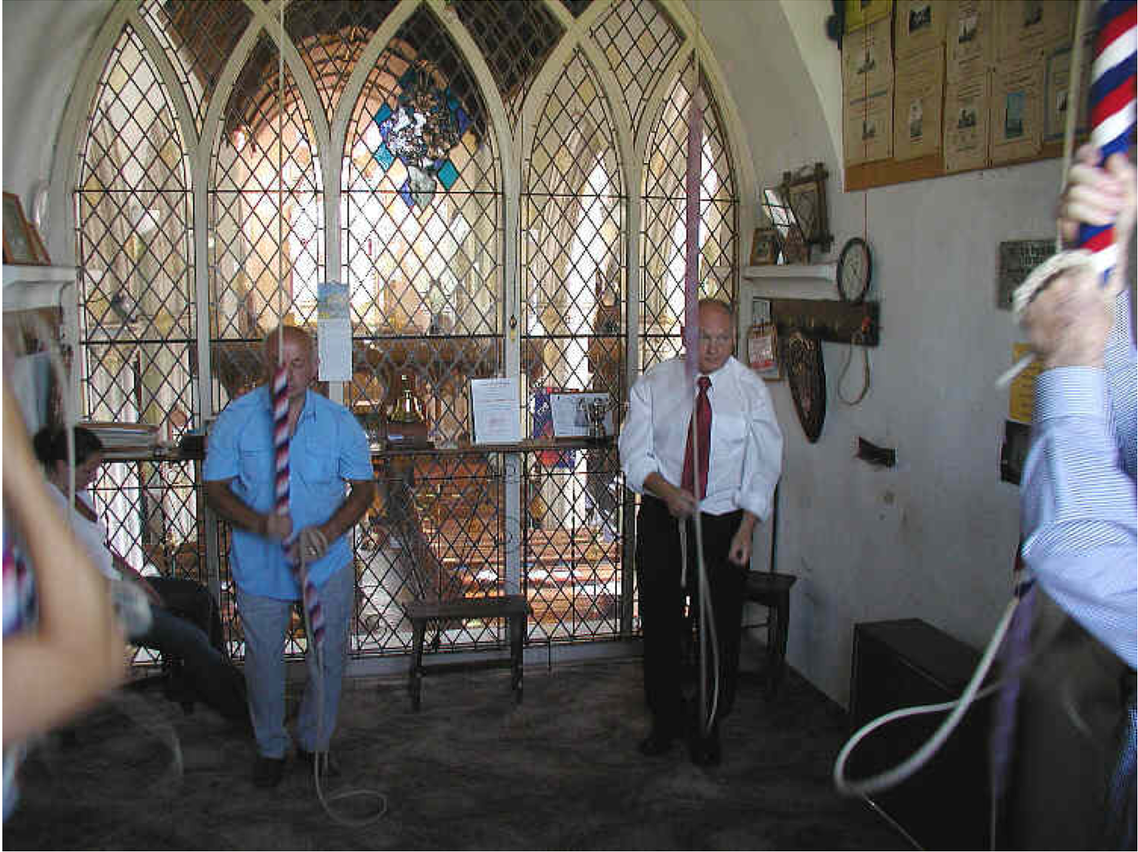These are the bells of St Bees Priory shown in the up position. When rung, they swing through a full circle from mouth upward around to mouth upward, and then back again.A typical tower has eight bells, ranging in pitch from the treble (bell #1) to the tenor (#8). When rung in the natural order 12345678 (called rounds), the tones form a descending major scale. Ringing methods require ringing the eight bells in the order corresponding to a permutation of {1, 2, 3, …, 8} and continuing through dozens to thousands of such “bars” of music (I will call each bar a row). The essential rules are:

1. The first row 12345678 is usually played twice to establish rhythm and parity, but will be given only once in the work here.
2. A row must never repeat a previous row, except that the final row is the same as the first. This property is called truth.
3. A bell can never move more than one position from its current position.

Rule 3 is a consequence of the physical nature of the wheels. It takes time for the wheel to rotate, and proper rhythm means that a bell cannot move far from one row to the next: it either stays in place (called making places) or moves one place to the right or left.

To see and hear more about how this works in a bell tower, watch these YouTube videos:

Methods have been developed so that—in the case of seven bells, for example—a sequence of 5,040 permutations can be rung (from memory: no written notes), starting and ending with rounds and never otherwise repeating a row. Really there are 5,041 permutations, but the number of changes—the moves from one row to the next—is 5,040. This is called a full extent on seven bells, as all 7! permutations appear. A full extent on eight bells has 40,320 changes, too many to be practical.

Any sequence of changes numbering at least 5,000 is called a peal (a quarter-peal has at least 1,250 changes). Ringing a peal or quarter-peal is a significant mental and physical challenge, and it is remarkable that methods have been developed that allow such things to be done from memory.

Before getting to the code demonstrating different aspects of bell ringing in general and Wolf Wrap in particular, however, we must evaluate the following cell to run the necessary initialization code: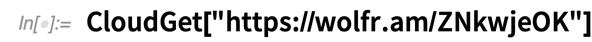✕

Now, consider the method shown in the following grid: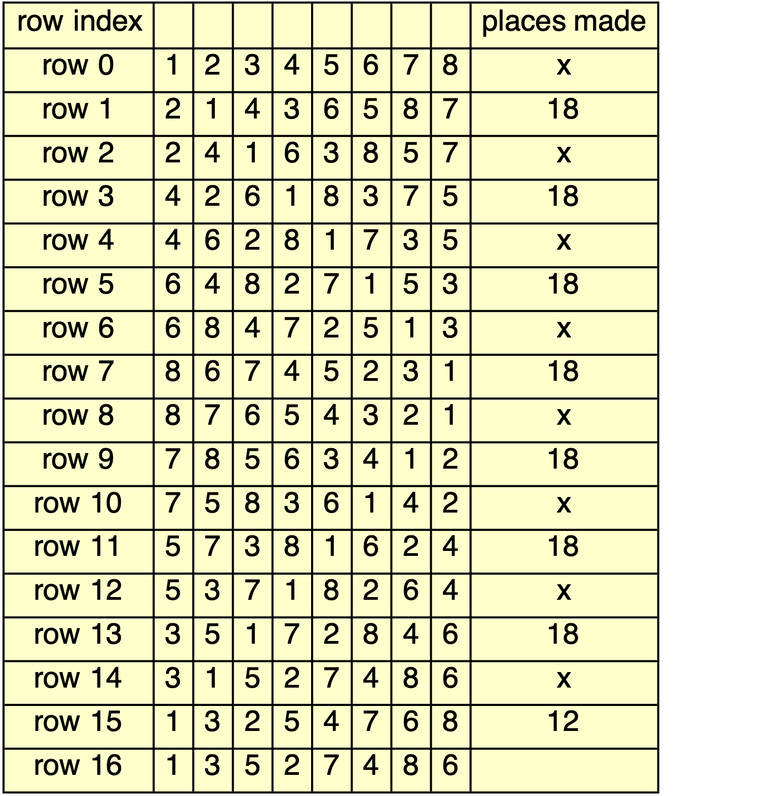✕

The three rules are obeyed and the last row is 13527486. Note how the treble bell (#1) moves up from position 1 to position 8 and then back to position 1. The other bells do the same (except for the last change) but with an offset. This pattern is easy for the ringers to remember.

This is a permutation of order 7—the 7-cycle (2, 3, 5, 7, 8, 6, 4):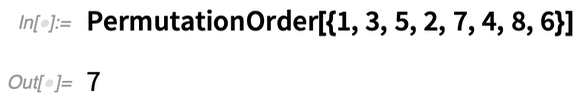✕

In the first change in the preceding grid, no bell makes places; that is denoted by ×. At the second change, places are made at positions 1 (by bell 2) and 8 (by bell 7). So the place notation for the sequence above is ×18×18×18×18×18×18×18×12. A ringer would learn this method by memorizing, for each change, the positions at which bells make places (i.e. the bell does not change its position). The previous grid represents the first lead of plain Bob major; “major” indicates eight bells. We will use {} for the ×; thus: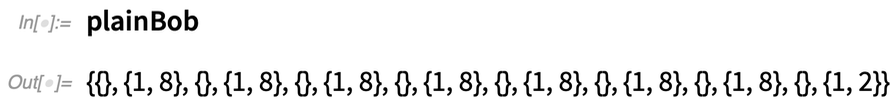✕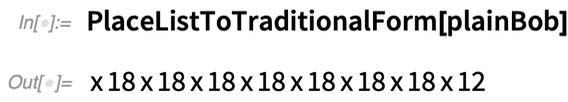✕

Here is what plain Bob major sounds like. Note the pleasing musical pattern at row 8, which is the reverse of the identity, called back rounds. There is a small pause after every even-indexed row, which is standard practice: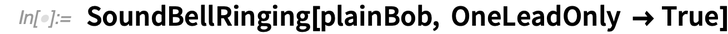✕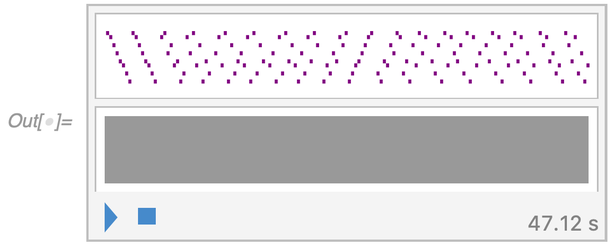If the place notation is simply repeated seven times, then the final row will be rounds. That is called a plain course of plain Bob major. Here the plain course has seven leads. The permutation that starts each lead is called a lead-head. In the earlier grid, row 16 is the second lead-head; the second lead-head will play an important role in the constraints in §3. There are 7 ⋅ 16, or 112, changes and the plain course sounds like this: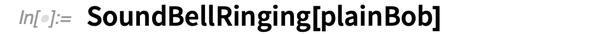✕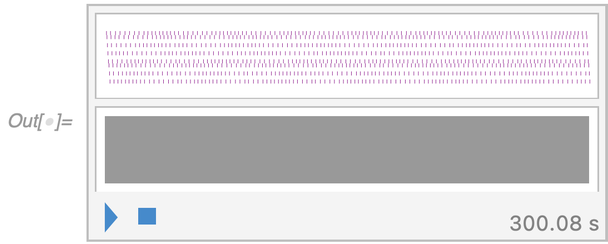Ringing a peal or quarter-peal is a complex physical and mental exercise: no written music is allowed, so it is possible only because, over several centuries, the ringers developed a concise system for memorizing the patterns that guide the bells. The ringers learn the place notation for the method and the conductor (one of the ringers) will call out certain special changes to be rung at the end of some leads. So while a plain course of plain Bob major has only 112 changes, the special changes allow this to be extended through 5,000 changes with no repetitions.

Rounds—the identity permutation 12345678—are pleasing to listen to but can occur only at the start and finish of a plain course. Mark Davies has devised methods that have several occurrences of rounds, but wrapping around the end of one change to the beginning of the next (this is called a wrap). The following two rows illustrate a wrap:

46587123
45678213

Here, 12345678 occurs at the end of the first row and the beginning of the second. An additional condition is that there should be no pause during the wrap. This means that a wrap should always start in an even-indexed row, using the indexing of the previous grid. My idea was to use ILP to develop a method that maximizes the number of wraps. ILP is available in Mathematica through the LinearOptimization function.

## 2. A Linear Description of Bell Ringing

To use ILP to invent a new ringing method, we need linear equations and inequalities to represent the basic rules. The starting point is to use variables B(x, y, i) with the conditions 0 ≤ B(x, y, i) ≤ 1 and B(x, y, i) ∊ ℤ. Such a B is called a binary variable: it is 0 or 1. The idea is that a value of 1 for B(x, y, i) means that bell i occurs at grid square (x, y), where y is a row number as indicated in the table of §1. We will restrict to eight bells and use k to denote the number of rows. In what follows, x and i will always vary from 1 to 8. First we set up the variables: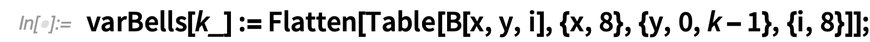✕

Define constraints, forcing each variable to be 0 or 1 and the first row to be rounds. Also enforce the condition that exactly one bell occupies any position and that bells in any row are a permutation of 1 through 8: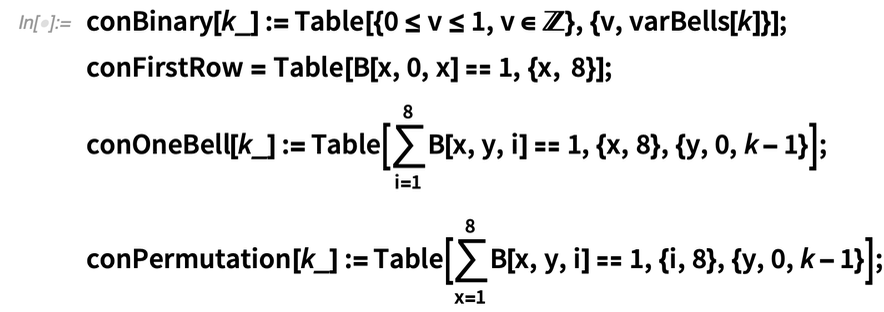✕

To enforce the critical bell rule 3, we eliminate the possibility of any bell moving more than one position. For example, B(3, 10, 1) + B(5, 11, 1) ≤ 1 says that bell 1 cannot move from position 3 in row 10 to position 5 in row 11: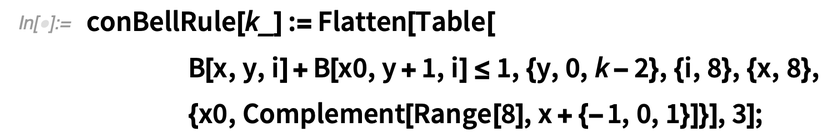✕

Place notation plays an important role later, so we show how to force a binary variable P(x, y, i) to indicate whether bell i makes places at position (x, y) (i.e. B(x, y, i) = B(x, y + 1, i) = 1). A natural approach is to set P(x, y, i ) = B(x, y, i) ⋅ B(x, y + 1, i), but this is illegal because multiplication is not a linear operation. There is, however, a standard technique in linear programming to reduce such a multiplication to four linear inequalities! I’m grateful to ILP expert Rob Pratt for teaching me this. First, here are the variables, including P(x, y), which indicate whether any bell makes places at the location. A simple sum guarantees that P(x, y) is as desired: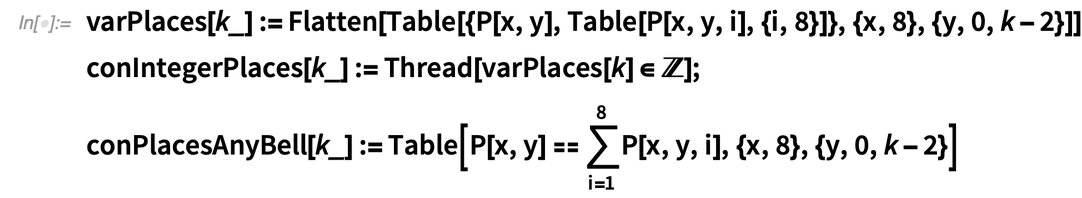✕

Here is the multiplication trick: four inequalities can be used to force P(x, y, i) to be the product of B(x, y, i) and B(x, y + 1, i). The identity is easily checked by running through the four possibilities for the two B values: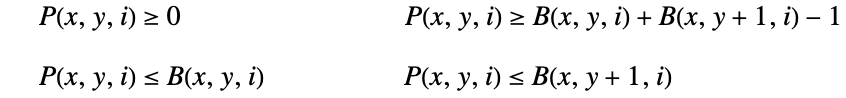This technique works in a similar way for products of more symbols, and that will be used later in the constraint that counts wraps: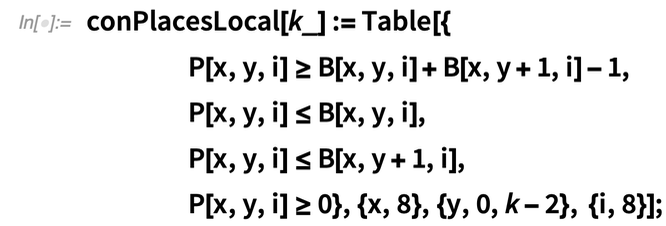✕

Another commonly enforced condition states that a bell cannot stay in its place three times in a row. This is called no long places. It is easy to set this up in linear fashion using the P variable: P(x, y) and P(x, y + 1) should not both be 1. We work modulo k − 1 so that we can compare the last-place list with the first, as that is relevant: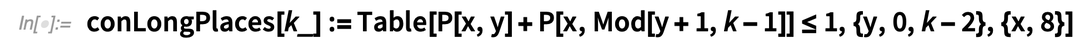✕

Truth is tricky and will be discussed later. One subterfuge that often leads to truth is to ask that the place notation does not have ×× and also that it never happens that places are made everywhere: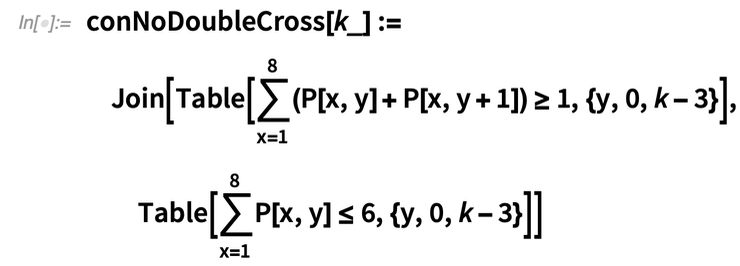✕

Here is a check. We use LinearOptimization with objective 0; this means it will search for an assignment of values that satisfies all the constraints. A key point is that for an optimization or feasibility problem with integer variables and linear constraints, LinearOptimization uses ILP. By default, it uses the ILP algorithms of the COIN-OR library. One can also (via the Method function) use the newer and state-of-the-art ILP solver called GUROBI, but a GUROBI license—it’s free for academic use or you can sign up for a free trial—is required.

The following search for a legal method takes well under a second with GUROBI, while the default method takes about 50 seconds: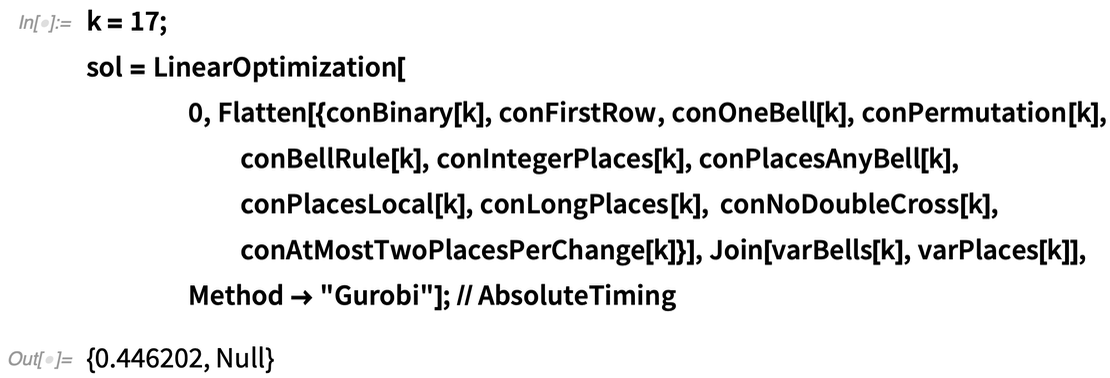✕

Here is what LinearOptimization found: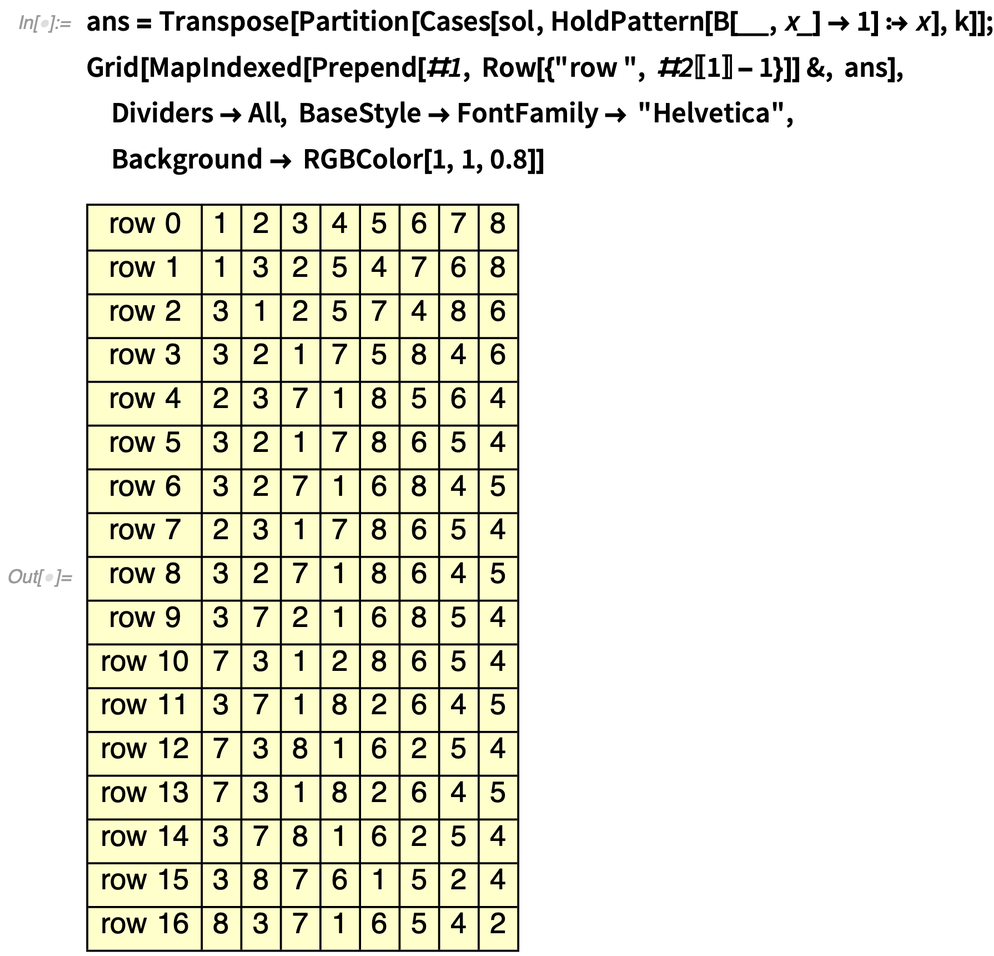✕

There are no repetitions in the rows, so the conNoDoubleCross constraint worked. Here is the place notation for the preceding; dots are used to separate data for rows when there is no ×: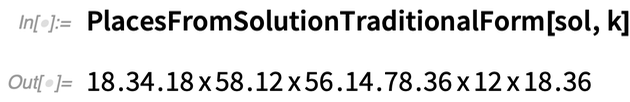✕

The last permutation has order 6, so the place notation for the 49-row plain course would consist of six repeats of the place notation: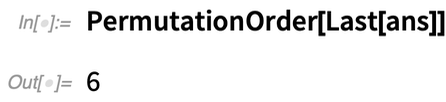✕

To skip to the chase, §3 will show how ILP can lead to the discovery of the method described by the following sequence of places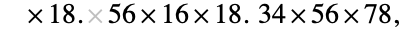which I called Wolf Wrap. The final permutation is 81234567, which is an 8-cycle (so the plain course has 8 ⋅ 12 = 96 changes) and Wolf Wrap has an unusual property that is of interest to the bell-ringing community: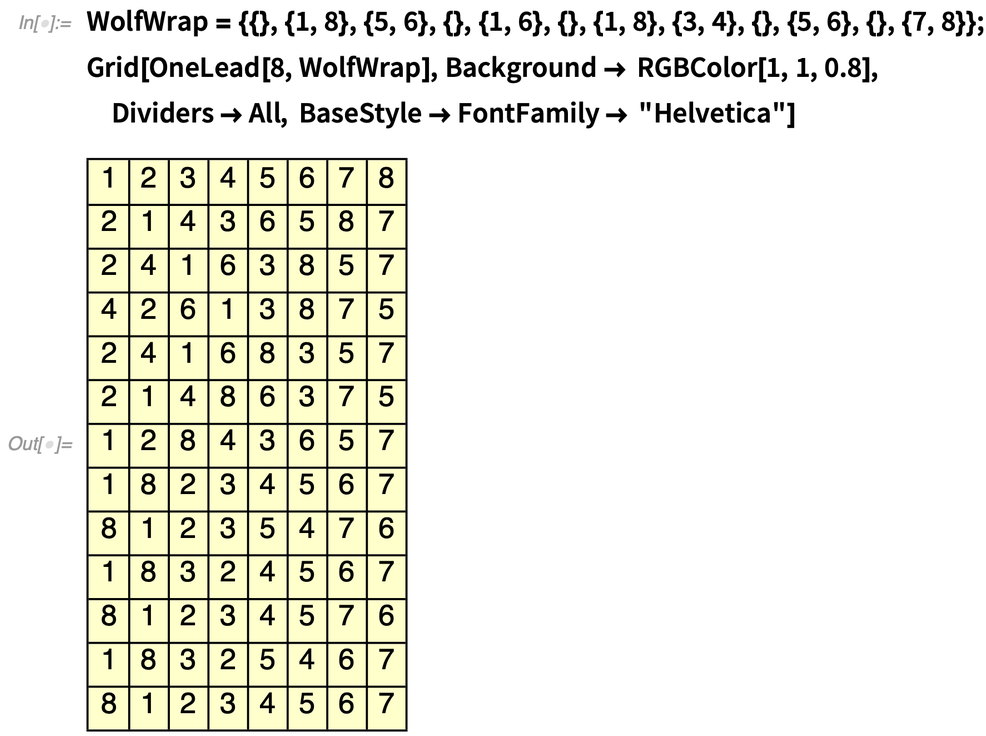✕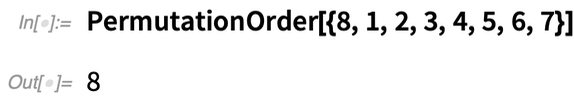✕

In the wider ringing world, methods often have some symmetry properties. We will not discuss those here; see  for more on symmetry and how to implement symmetry constraints linearly.

### Example: COIN-OR

Simple examples do not require GUROBI. The default method, COIN-OR, is used here on the example from this section. It is more than one hundred times slower: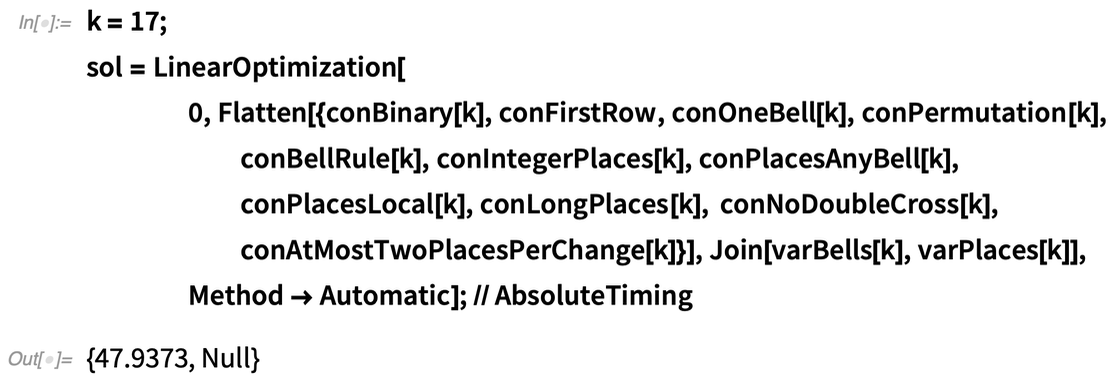✕

## 3. Wrapping It Up, Truthfully

Our goal is to obtain a method whose plain course has many wraps (and satisfies the truth condition), but we also want the method to have aesthetic and musical appeal. The latter is needed so a band will ring the method; having a successful ring of a quarter-peal or peal is a requirement for a method to become officially named and listed.

To set up an ILP search for a wrap-rich method, we must set up variables to count the number of wraps and also enforce truth (no repeated rows) for the method. These are both a little tricky. Some easier additional constraints are the following; they lead to place notation that is nicely simple for the ringers to memorize:

1. The number of places made at each change should be either 0 or 2.
2. The total number of places made in the entire method should not be too large.

These have simple linear implementations: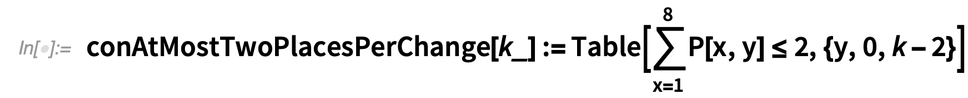✕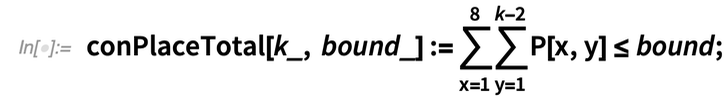✕

### 3.1. Counting Wraps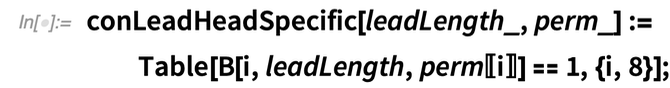✕

Recall that in the constraint for places, we made use of a subtle product construction: one can set one binary variable to be the product of two other binary variables via four inequalities. This generalizes to M binary variables as opposed to just two by using 2M inequalities. To get the product W of V1, V2, …, VM, use 0 ≤ W, WVi, W ≥ 1 − M + ΣVi.

So we can introduce W(x, y, p) to specify that a wrap starts at position (x, y + jL), where x runs from 2 to 8 (1 is excluded because rounds can appear only once), y runs from 1 to 11 in steps of two (because of the parity issue) and p is one of the eight powers of the lead-head permutation. Using the powers in this way provides a method for dealing with a property that can occur in any of the eight leads. The wrap constraint is then the product

W(x, y, p) = B(x, y, p1) ⋅…⋅ B(8, y, p8 – x + 1) ⋅ B(1, y + 1, p8 − x + 2)… ⋅ B(x − 1, y + 1, p8),

where the product trick is used to express this using 2 ⋅ 8 = 16 inequalities. If this product is 1 and p is the jth power (j = 0, …, 7), then the specified locations have bells 1 through 8 in order, and there is a wrap starting in the (y + jL)th row, starting at position x. The full code for the wrapping and truth constraints is in the supplement.

Recalling the parity issue concerning wrap, the following constraint will then force the number of wraps to equal the target where the initial row of rounds counts as a wrap. This assumes that the lead length is even; it is a little different in the odd case: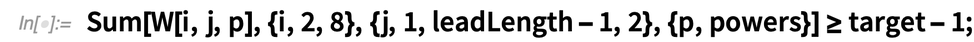✕

### 3.2. Enforcing Truth

Truth will be handled in a way similar to the wrap count. We will specify the lead-head and then say that the jth row in the first lead is not equal to the mth row in another lead (mj), using powers of the lead-head as was done for wraps. First, consider how to compare two rows in the first lead. B(x, y1, i) + B(x, y2, i) − 2B(x, y1, i) ⋅ B(x, y2, i) = 1 means that the x position in the two rows are different as regards bell i; a value of 0 means they are the same. So we can simply say that the sum of B(x, y1, i) + B(x, y2, i) − 2B(x, y1, i) ⋅ B(x, y2, i) over all choices of x and i must be at least 1. Of course, we use the product trick discussed earlier (use a new binary variable for each possible product) to linearize the products that arise. And to deal with rows in different leads, we use the permutation that forms the lead-head, which we take as known for our application.

### 3.3. Discovering Wolf Wrap

So here is the final code to find a method having nine wraps. The lines starting with If[EvenQ[leadLength] where target–1 appears account for the fact that the occurrence of rounds in the first row is considered to be a wrap (it is a legal occurrence of rounds). That line uses the parity of the lead length to choose the y values where a wrap is legal. The parameters here—nine for the target number of wraps and 14 for the total number of places—were obtained after some trial and error led to the conclusion that these values were optimal: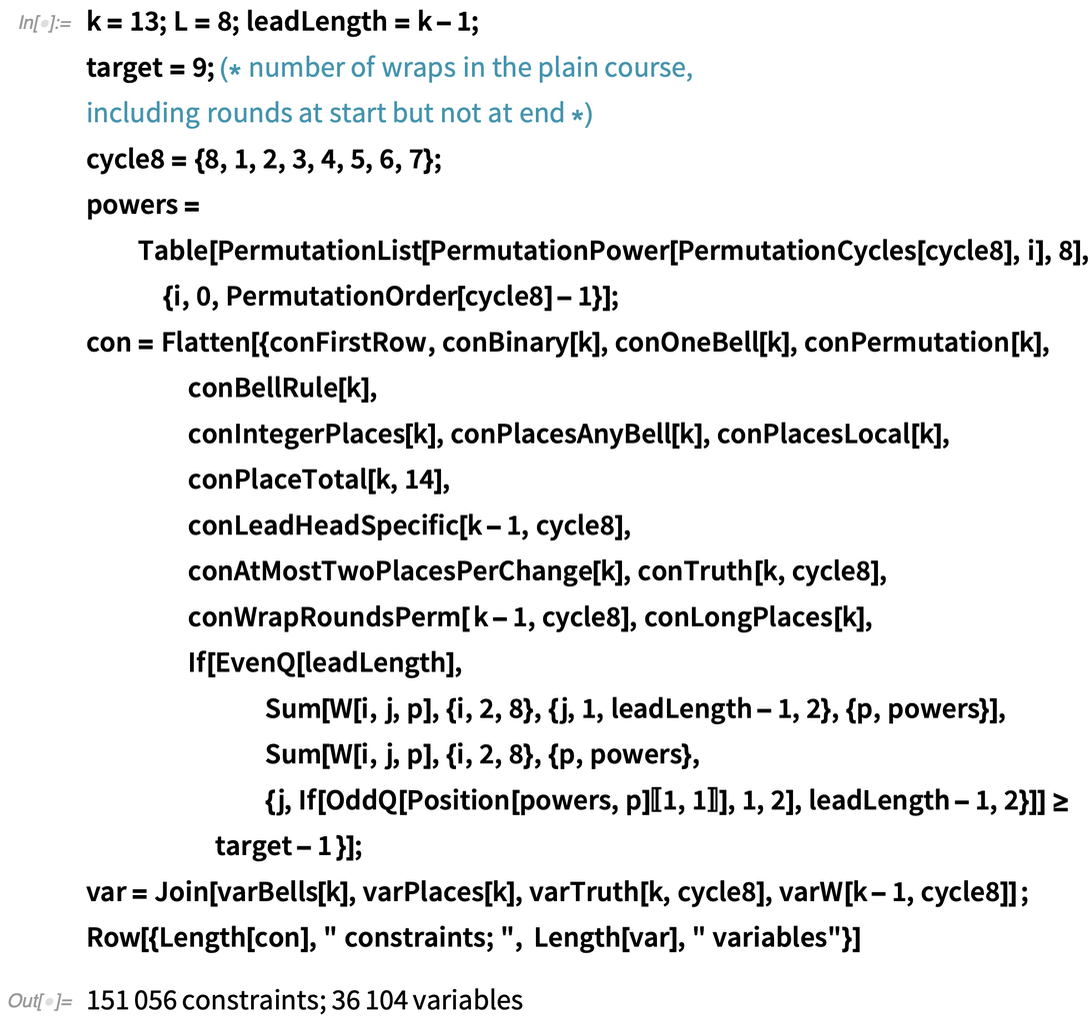✕

The resulting linear system is enormous, with over 36,000 variables and over 151,000 constraints. It is remarkable that GUROBI finds a solution in about three minutes: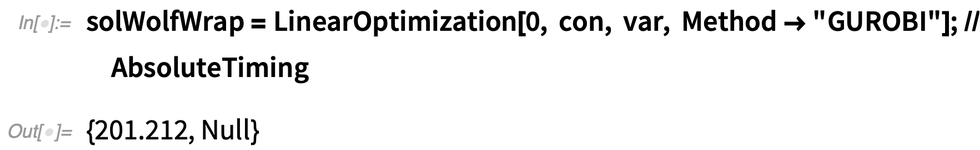✕

Here is what this method, which I named Wolf Wrap, looks like: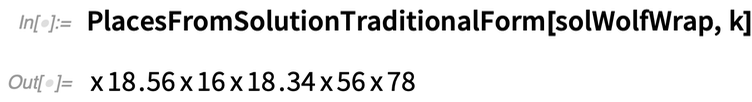✕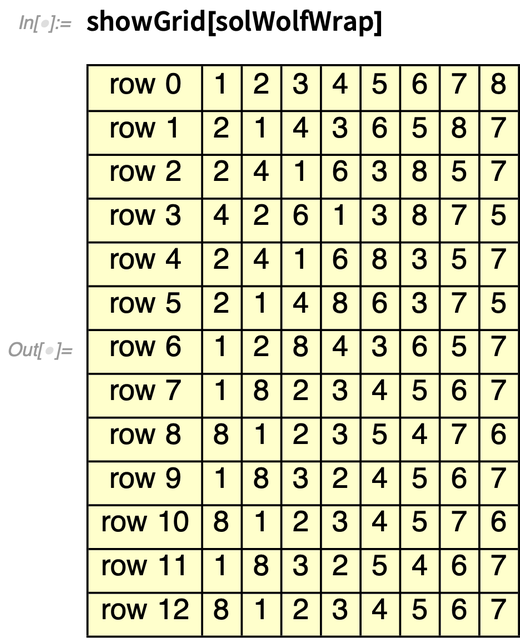✕

Here is the plain course for Wolf Wrap with the wraps shown in bold, red numbers: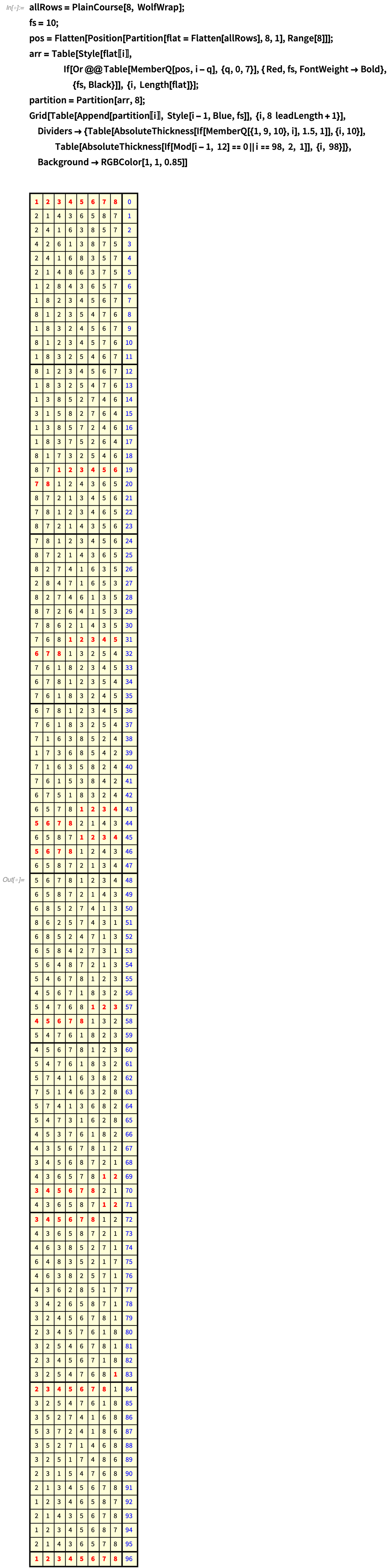✕

And here is what it sounds like: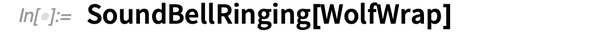✕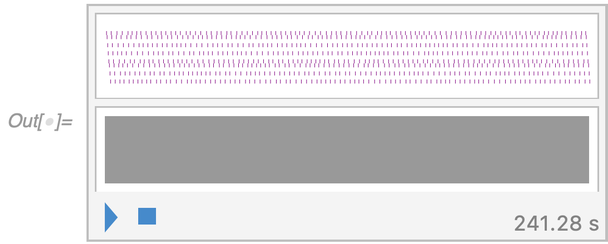The plain course has 96 rows. To turn this into a quarter-peal, switches at various lead-ends must be introduced. Such a composition was constructed by Janet Archibald, who spliced Wolf Wrap with the Stedman triples method. Her composition of a quarter-peal was then rung on June 3, 2021, in just under an hour; see the Ringing Room and Composition Library.

There were 1,272 changes, so the average ringing time for each bell was was 56 ⋅ 60 ∕ (1272 ⋅ ((8 + ½)), or 0.31 seconds. (The ½ is because of the pause that occurs after every two changes.) I am grateful that Mark Davies, who liked the method, asked Janet Archibald to turn it into a quarter-peal composition, and both were members of the band that rang the quarter-peal.

## 4. ILP Is Awesome

The diversity of problems that can be attacked with ILP is immense because binary variables can be applied to so many optimization problems. I use it in a consulting business where incoming college students present their preferred courses in order, and we assign them to classes so as to optimize the choices from the students’ perspectives. ILP is also used to make up the schedules for professional sports leagues, and there are myriad other applications. Mathematica makes it easy to set up an ILP, and we can now add bell ringing to the list of endeavors where ILP can play a role.